# Thermal Performance of a 4-stroke EngineA four-stroke engine is a piston engine with a stroke for two revolutions of the crankshaft. With a four-stroke diesel engine, the air is compressed during the compression stroke, heating it. At the end of the compression stroke, the fuel is injected through an injector and spontaneous combustion occurs. The expanding combustion gases push the piston down during the work stroke, which drives the engine.

The 4-stroke engines can be Otto cycle or Diesel cycle. However, for performance purposes we analyze them together.

Explosion engines take advantage of the chemical energy of the fuel (gasoline, diesel, etc.). At the time of the explosion this energy becomes thermal energy. Thanks to the different elements of the motor, this thermal energy is converted into mechanical energy (in this case kinetic energy) by rotating an axis.

Performance is the ratio of energy that is used in these transformations. According to the laws of thermodynamics, it can never exceed 1 in a theoretical model. In a real cycle, the practice can never reach 1.

According to the second law of thermodynamics, no real or ideal motor can turn all introduced heat into mechanical work. Therefore, only a fraction of the heat supplied by combustion will be transformed into work; This fraction represents the thermal performance of the engine.

Then we define, ideal thermal performance to the relationship between the amount of heat transformed into useful work and the amount of heat supplied to the fluid.As the useful work is equivalent to the difference between the heat supplied Q 1  and the heat subtracted Q 2 we have:

Anyone can form a more complete idea of ​​the concept of thermal efficiency and become familiar with the interpretation of the diagrams by examining the sequence of transformations in PV and TS coordinates for an ideal cycle.

## Thermal Performance in the Compression Phase

The yellow zone (L2) represents the work done by the piston. The piston moves from the bOttom dead center to the top dead center.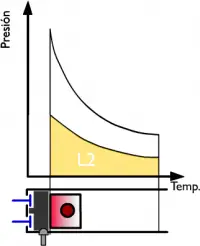## Thermal Performance in the Combustion Phase of a 4-stroke Engine

The heat produced by combustion is instantaneously introduced, since the cycle is at constant volume. The blue zone QI represents the heat supplied.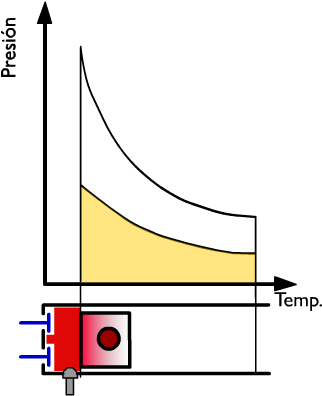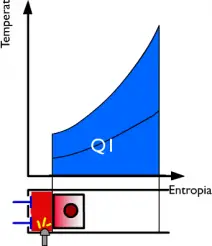## Thermal Performance in the Expansion Phase of a Thermal Engine

The piston moves, by default, from the fluid pressure, from the top dead center to the bOttom dead center. The LI work produced by the active fluid during the expansion is represented in PV coordinates, by the striped area between the upper adiabatic expansion line and the axis of the abscissa.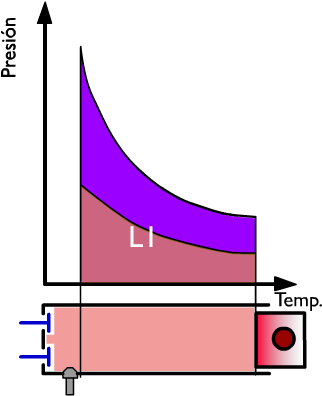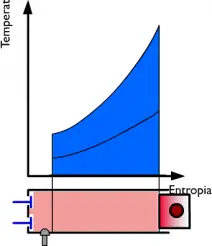## Thermal Performance in the Gas Exhaust Phase

At this moment the exhaust at constant volume ensues and during this phase of the cycle the heat Q2 is subtracted while the pressure drops. On the right side, the heat Q is represented, in coordinates TS, by the dark blue surface. In violet the useful work is represented and in light blue the heat used.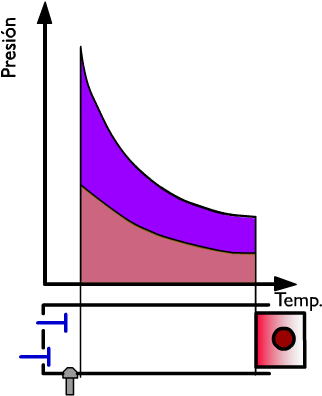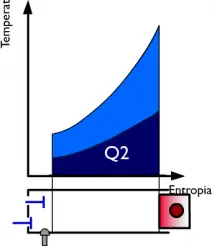As the useful work, obviously, equal to the heat used, we can write: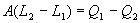in this way we can simplify the calculation of the useful work and the thermal efficiency of the cycle using the values ​​Q 1  and Q 2  instead of L 1  and L 2 .

Author:

Published: August 29, 2012
Last review: February 18, 2020